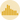# Basic histogram with Seaborn

Histograms are used to display the distribution of one or several numerical variables. Seaborn enables us to plot both the histogram bars as well as a density curve obtained the same way than kdeplots.

With Seaborn, histograms are made using the histplot function. You can call the function with default values, what already gives a nice chart. Though, do not forget to play with the number of bins using the ‘bins’ argument. Indeed, a pattern can be hidden under the hood that one would not be able to detect with default bins values.

## Drawing a simple histogram with default parameters

``````# libraries & dataset
import seaborn as sns
import matplotlib.pyplot as plt
# set a grey background (use sns.set_theme() if seaborn version 0.11.0 or above)
sns.set(style="darkgrid")
sns

sns.histplot(data=df, x="sepal_length")
plt.show()``````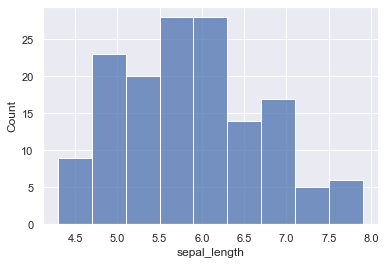You can add a kde curve to a histogram by setting the `kde` argument to True.

``````# libraries & dataset
import seaborn as sns
import matplotlib.pyplot as plt
# set a grey background (use sns.set_theme() if seaborn version 0.11.0 or above)
sns.set(style="darkgrid")

sns.histplot(data=df, x="sepal_length", kde=True)
plt.show()``````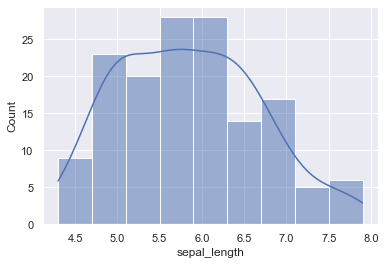Another way of drawing a histogram with Seaborn is by using the distplot function. In versions before 0.11.0, it automatically added a kdeplot-like smooth curve. Note that this function will be deprecated soon. Refer to the new distplot function documentation for future use.

``````# libraries & dataset
import seaborn as sns
import matplotlib.pyplot as plt
# set a grey background (use sns.set_theme() if seaborn version 0.11.0 or above)
sns.set(style="darkgrid")

sns.distplot(df["sepal_length"])
# in the next version of the distplot function, one would have to write:
# sns.distplot(data=df, x="sepal_length", kind='hist') # note that 'kind' is 'hist' by default
plt.show()``````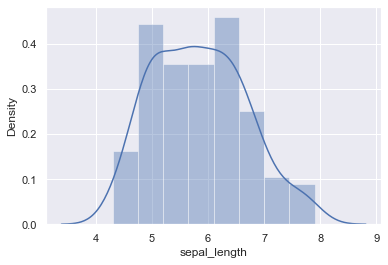## Controlling for the number of bins

``````# libraries & dataset
import seaborn as sns
import matplotlib.pyplot as plt
# set a grey background (use sns.set_theme() if seaborn version 0.11.0 or above)
sns.set(style="darkgrid")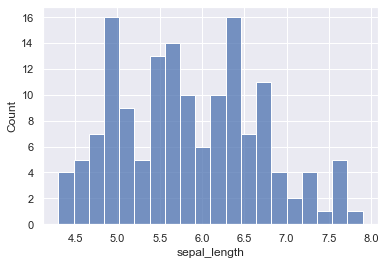👋 This document is a work by Yan Holtz. Any feedback is highly encouraged. You can fill an issue on Github, drop me a message onTwitter, or send an email pasting `yan.holtz.data` with `gmail.com`.